We all have plotted line graphs on coordinate planes (X-axis and Y-axis) before. Where x variable is an independent variable, usually called a predictor variable and y is a dependent variable, called the criterion variable. To predict the relationship between these two variables, regression analysis is used.

For instance, you are aware of the fact that a house of 2,200 square feet costs 50,000$. You wish to buy a 3,500 square feet house. How much it will cost you? In order to find the price, you need a relationship between price and the area of the house. Linear regression solves helps you do this. Let’s find out! Simple Linear Regression Simple linear regression is the simplest approach for statistical learning. It is a part of Bivariate Statistics. Bivariate meaning, two variables. Now the relationship between variable 1 and variable 2 is special in case of Linear regression. Thus, the value of one variable is a function of the other variable.$y = f(x)$ The first equation which crosses our minds after reading this is the equation of the line. The slope-intercept form of a line:$y=m*x+b$ In this formula, x is an independent variable, y is a dependent variable along with two real components, m, and b. Where, m is the slope of the line and b is the y-intercept that is when the line crosses the Y-axis i.e. value of y when x=0. Now, let’s take an example:$y=2*x+3$ We can learn a lot about this equation by superimposing this equation on the slope-intercept form of the line equation. The slope of the line = 2 Y-intercept = 2(0)+3 = 3 On plotting, it gives a straight line-We had a look at the algebric formula of lines and now we will examine its connection with linear regression. Linear Regression Equation: Consider the following dataset in Table-1, which has prices of houses based on the area of each house. Area (in square feet) Price (in thousand$)
1500
340
1750
390
2600
550
3000
565
3200
610
3600
680
4000
725
2000
490

Now, with the help of the data provided in the table, we have to predict the price of the house that has an area of 3,500 square feet.

Step – 1: We will plot a scatter graph using the available data points.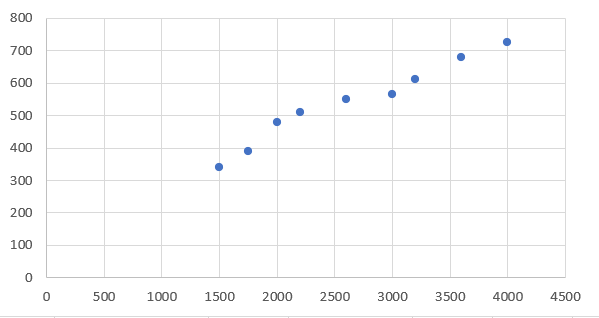Note: The order in which data points are plotted doesn’t matter. We can graph the points in any order. This just happens to be the final graph we ended up with.

Step – 2: Look for a rough visual line.Warning: If you try and find a linear regression equation through an automated program like Excel, you will find a solution, but it does not necessarily mean the equation is a good fit for your data.

As you can see, there are several lines passing through the data points. We don’t know that any one of these lines here is the actual regression line. So which line is it? To answer this question, let’s get to the next step.

Step – 3: In this step, descriptive statistics is done in order to find the ‘best-fit line. We will start by finding the mean of each variable.

The formula for finding the mean-$Mean = \frac{Sum of Observation}{Total number of Observations}$

Mean for Area = xm = 2706.25 square feet

Mean for House prices = ym = 543.75 $Plot this point, (xm,ym) in the graph. This point is termed the centroid.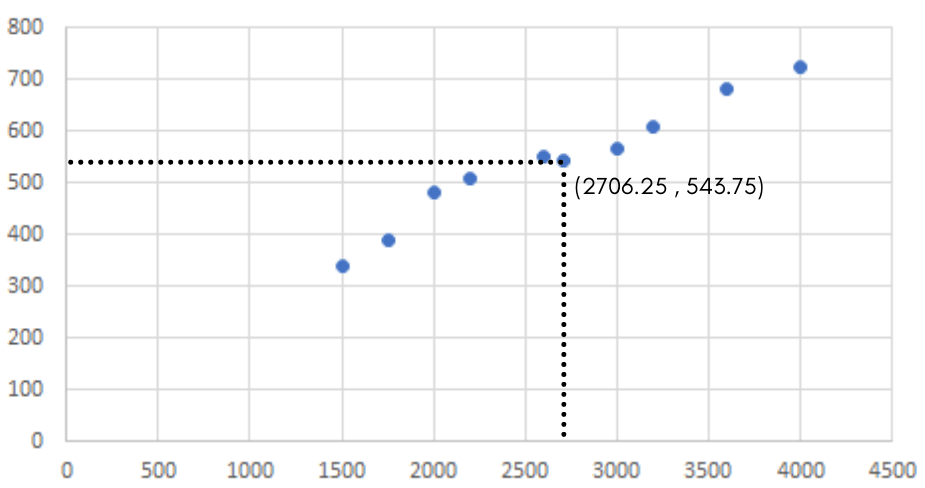Note: The best-fit regression line must pass through the centroid, which comprises the mean of x variable and mean of y variable. Step – 4: To make the best-fit line we need two points. The centroid gives you one point to work with. Let’s move ahead with the calculations. Mathematically, the regression line shares the characteristics of being linear with adjustable parameters. Remember the general equation we learned of the line, the slope formula.$y = mx+b$ where m is the slope of the line and b is the y-intercept. Finding for finding slope-$m=\frac{\displaystyle\sum_{i=1}^{n}(x - xm) (y - ym)} {\sum_{i=1}^{n}(x - xm)^2}$ Finding the value of y-intercept b, we take ym which is the mean of the dependent variable and then subtract the slope times the mean of the independent variable.$b=ym-m*(xm)$ We already calculated values of xm and ym earlier in Step – 3. Calculations: In order to form our linear equation, each data point should be considered for calculating slope. So let’s re-create our Table-1 by adding the necessary columns. Area (sq. feet)$x$ Price ($)$y$
Area Deviation$(x - xm)$
Price Deviation$(y - ym)$
Deviation Product$(x - xm)(y - ym)$
Area Deviation squared$(x - xm)^2$
1500
340
-1206.25
-203.75
245773.4375
1455039.063
1750
390
-956.25
-153.75
147023.4375
914414.0625
2000
490
-706.25
-53.75
37960.9375
498789.0625
2600
550
-106.25
6.25
-664.0625
11289.0625
3000
565
293.75
21.25
6242.1875
86289.0625
3200
610
493.75
66.25
32710.9375
243789.0625
3600
680
893.75
136.25
121773.4375
798789.0625
4000
725
1293.75
181.25
234492.1875
1673789.063

Note: Regression is very sensitive to rounding. Thus, it is best to take your calculations to four decimal places.

From the table, we can say-

Sum of deviation product =$\displaystyle\sum_{i=1}^n(x - xm)(y - ym)$ = 825312.5

Sum of area deviation squared =$\displaystyle\sum_{i=1}^n(x - xm)^2$ = 5682187.5

Slope = m=$\frac{\displaystyle\sum_{i=1}^{n}(x - xm) (y - ym)} {\sum_{i=1}^{n}(x - xm)^2}$ =$\frac{825312.5}{5682187.5}$ = 0.14526

We found the values xm, ym, m and thus y-intercept is$b=ym-m*(xm)$ =$543.75 - 0.14526 * 2706.25$ = 150.64

Now, as our final step, we will assemble the values of slope and y-intercept gives us the following regression equation.$y=0.14526*x+150.64$

Remember we discussed that our centroid has to fall on the best-fit regression line. Well, it does.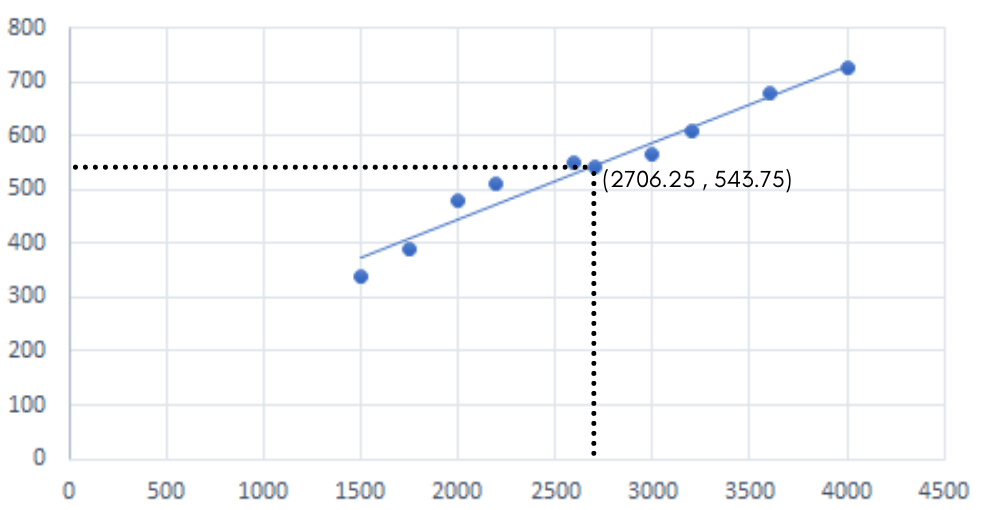This method is The Least Squares method.

Interpretation: For every 1 square foot the area increases, we would expect the price to increase by 0.14526 thousand $that is, 145.26$.

Implementation using Kotlin

You are now aware of the concept of linear regression. Let’s start with the implementation of what we learned.

Dataset-

We will be using a self-generated dataset. This is just an arbitrary choice, you can use any other dataset of your choice. The first step is splitting the data between testing and training data sets.

The training process builds up the machine learning algorithm. This data is fed to the algorithm, the model evaluates the data repeatedly to learn about the behavior of data and then reveals patterns to serve the intended purpose.

After the model is built, testing data validates that it can make accurate predictions. The testing data should be unlabeled. It acts as a real-world check of an unseen dataset to confirm that the algorithm is trained effectively.

We usually split the data around 20%-80% between the testing and training stages.

Code-

Starting with the training dataset representing salary in thousands per year of experience.

				
val xs = arrayListOf(1, 2, 3, 4, 5, 6, 7, 8, 9, 10) //independent variable
val ys = arrayListOf(25, 35, 49, 60, 75, 90, 115, 130, 150, 200) //dependent variable




As discussed earlier, the next step is to find the centroid. That is the mean of both variables.

				
val meanX = xs.average() //Mean of independent variable
println(meanX) //print function



Output: 5.5

				
val meanY = ys.average() //Mean of dependent variable
println(meanY) //print function



Output: 92.9

The coordinates of the centroid are (5.5, 92.9). Let’s move forward!

Training the model-

				
val yearsdeviation = xs.map{it - 5.5} //Finding the deviation of years
//map function is an inbuilt function whih applies the specified condition to every element of list
println(yearsdeviation)



Output: [-4.5, -3.5, -2.5, -1.5, -0.5, 0.5, 1.5, 2.5, 3.5, 4.5]

				
val salarydeviation = ys.map{it - 92.9} //Finding deviation in the salary
println(salarydeviation)



Output: [-67.9, -57.900000000000006, -43.900000000000006, -32.900000000000006, -17.900000000000006, -2.9000000000000057, 22.099999999999994, 37.099999999999994, 57.099999999999994, 107.1]

				
val deviationproduct = xs.zip(ys) { x, y -&gt; (x - meanX) * (y - meanY) } //Multiplying both the list generated
//zip function merges two different lists into a new list
println(deviationproduct)



Output: [305.55, 202.65000000000003, 109.75000000000001, 49.35000000000001, 8.950000000000003, -1.4500000000000028, 33.14999999999999, 92.74999999999999, 199.84999999999997, 481.95]

				
val sqofyearsdeviation = area.map {e -&gt; e.pow(2)} //Squaring of deviation of years column
//e denotes each element in the list
println(sqofyearsdeviation)



Output: [20.25, 12.25, 6.25, 2.25, 0.25, 0.25, 2.25, 6.25, 12.25, 20.25]

				
val num = deviationproduct.sum() //summation of all elements in the list
println(num)



Output: 1482.5

				
val deno = sqofyearsdeviation.sum()
println(deno)



Output: 82.5

Now let’s calculate value of slope-

Slope =$\frac {num}{deno}$

				
val slope = num / deno
println(slope)




Output: 17.96969696969697

				
val slope:Double = String.format("%.3f", 17.96969696969697).toDouble() //Rounding off the value of slope
println(slope)



Output: 17.97

After calculating slope, the centroid is used to find Y intercept –

				
val yIntercept = meanY - slope * meanX // Finding value of Y Intercept
yIntercept




Output: -5.934999999999988

				
//Final equation of linear regression
val simpleLinearRegression = { independentVariable: Double -&gt; slope * independentVariable + yIntercept }



Our model is trained now. Let’s test it!

Testing the model-

For instance, how much an individual with 2.5 or 6.5 years of experience should be entitled to earn?

				
val testcaseone = simpleLinearRegression.invoke(2.5) // Predicting the salary of an employee with experience of 2.5 years
testcaseone



Output: 38.99000000000001

				
//Rounding off predicted value of test case 1
val testcaseone:Double = String.format("%.3f", 38.99000000000001).toDouble()
testcaseone



Output: 38.99

				
val testcasetwo = simpleLinearRegression.invoke(6.5) // Predicting salary of an emplyee with 6.5 years experience
testcasetwo



Output: 128.83999999999997

				
//Rounding off predicted value of test case 2
val testcasetwo:Double = String.format("%.3f", 128.83999999999997).toDouble()
testcasetwo



Output: 128.84

Our model predicted the salary with respect to years of experience of the employee. Now the question is, how accurate is this value? What is the error percentage between the original value and the predicted value? To answer these questions let’s move to the next section.

Building Regression Model in S2

S2/Kotlin has ample packages to solve linear model problems using regression models. We will implement the Ordinary Least Squares(OLS) model. To build and analyze a model for a given dataset,$LMProblem$ is constructed.

Q. Consider a response vector(dependent variables) as:$Y = \begin{bmatrix}1\\2\\4\\5\\10\end{bmatrix}$ and design matrix of explanatory vector(independent variables) as:$X = \begin{bmatrix}25\\35\\60\\75\\200\end{bmatrix}$. Construct a OLS model using S2 IDE.

				
%use s2 //a keyword used in every program of S2

//Defining the vector Y
val Y: Vector = DenseVector(arrayOf(1.0, 2.0, 4.0, 5.0, 10.0))

//Defining the matrix X
val X: Matrix = DenseMatrix(
arrayOf(
doubleArrayOf(25.0),
doubleArrayOf(35.0),
doubleArrayOf(60.0),
doubleArrayOf(75.0),
doubleArrayOf(200.0)
)
)

//estimation of true intercept
val intercept = true
val problem1 = LMProblem(Y, X, intercept)
printOLSResults(problem1) //calling the function

//Runs an OLS regression
//Block for defining the function
fun printOLSResults(problem: LMProblem?) {
val ols = OLSRegression(problem)
val olsResiduals: OLSResiduals = ols.residuals()
//coefficients for explanatory variables
println("beta hat: ${ols.beta().betaHat()}\nstderr:${ols.beta().stderr()},\nt: ${ols.beta().t()},\nresiduals:${olsResiduals.residuals()}")
//beta is the slope value
//Standard error is stderr
//Residual is the difference between original and model value
}



Output:

beta hat: [0.048918, 0.535481]

stderr: [0.005264, 0.532022] ,

t: [9.293036, 1.006500]

residuals: [-0.758430, -0.247609, 0.529441, 0.795672, -0.319074]

FIT of regression model

Till now we have covered and coded fundamental concepts of Simple Linear Regression. In this section, we will learn to evaluate how a regression line fits the data it models.

A regression model is unique to the data it represents. Once a regression model is built, the sum of squared errors or residuals is calculated using the regression line. Till now we have calculated the regression line using the Least Squares Method. Now, we will find the errors and residuals.

In statistics, the residual and error are not the same. Error is defined as the difference between the observed value and true value(unobserved value). Whereas, residual is defined as the difference between observed value and model value(predicted values).

Error Calculation:

Recall the example of house prices we considered. We already discussed and found the equation of linear regression on selected data and how the line passes through the centroid. Now let’s move on to the part where we calculate predicted prices for each house with the help of the equation we derived earlier.

Area (square feet)
Price (thousand $)$y=0.14526*x+150.64$$y$ (predicted prices) 1500 340$y=0.14526*(1500)+150.64$ 368.53 1750 390$y=0.14526*(1750)+150.64$ 404.845 2600 550$y=0.14526*(2600)+150.64$ 528.316 3000 565$y=0.14526*(3000)+150.64$ 586.42 3200 610$y=0.14526*(3200)+150.64$ 615.472 3600 680$y=0.14526*(3600)+150.64$ 673.576 4000 725$y=0.14526*(4000)+150.64$ 731.68 2000 490$y=0.14526*(2000)+150.64$ 441.16 All we are doing in the above table is to substitute each area value in place of x. After evaluating these equations, it will give us the predicted price in the last column. Basically in this case the point to be noted is that our training data and testing data are the same. Note: Instead of grabbing a calculator for the above calculations. Open S2 and code for each data point.   val y=0.14526*(1500)+150.64 //Calculating for first row println(y)   Output: 368.53 Observations: For a house of 1500 square feet, the price was 340 thousand$ and according to our regression equation we predicted 368.53 thousand $. Thus, you can observe the price of houses is not exactly the same as our predicted value. This discrepancy in values is what we refer to as an error. So let’s find the difference in the original price and the predicted price for all the data points we have. Area (square feet) Price (thousand$)$y$ (predicted prices)
Error = Observed – Predicted
Squared Error
1500
340
368.53
-28.53
813.9609
1750
390
404.845
-14.845
220.3740
2600
550
528.316
21.684
470.1958
3000
565
586.42
-21.42
458.8164
3200
610
615.472
-5.472
29.9428
3600
680
673.576
6.424
41.2678
4000
725
731.68
-6.68
44.6224
2000
490
441.16
48.84
2385.3456

Squaring the differences, the residuals come out as mentioned in the last column of the above table. On adding them up we get the Sum of Squared Errors (SSE).

Sum of Squared Error = SSE =$\displaystyle\sum_{i=1}^n(Observed-Predicted)^2$ = 4464.5257

Error in the scatter graph of Table -1 is the distance from the true line to each observed data point. Squaring all these errors (SSE) can be represented on the graph as –Residual Analysis:

Residual analysis is important to assess the appropriateness of a linear regression model. It is the second major step towards validating a model. If the model assumptions are not satisfied, residual analysis often suggests ways for improving the model in order to obtain better results.

For understanding residuals, recall the dataset and its scatter plot we studied in Table-1. Now, construct a parallel line to X-axis using the value of Ymean that is, 543.75.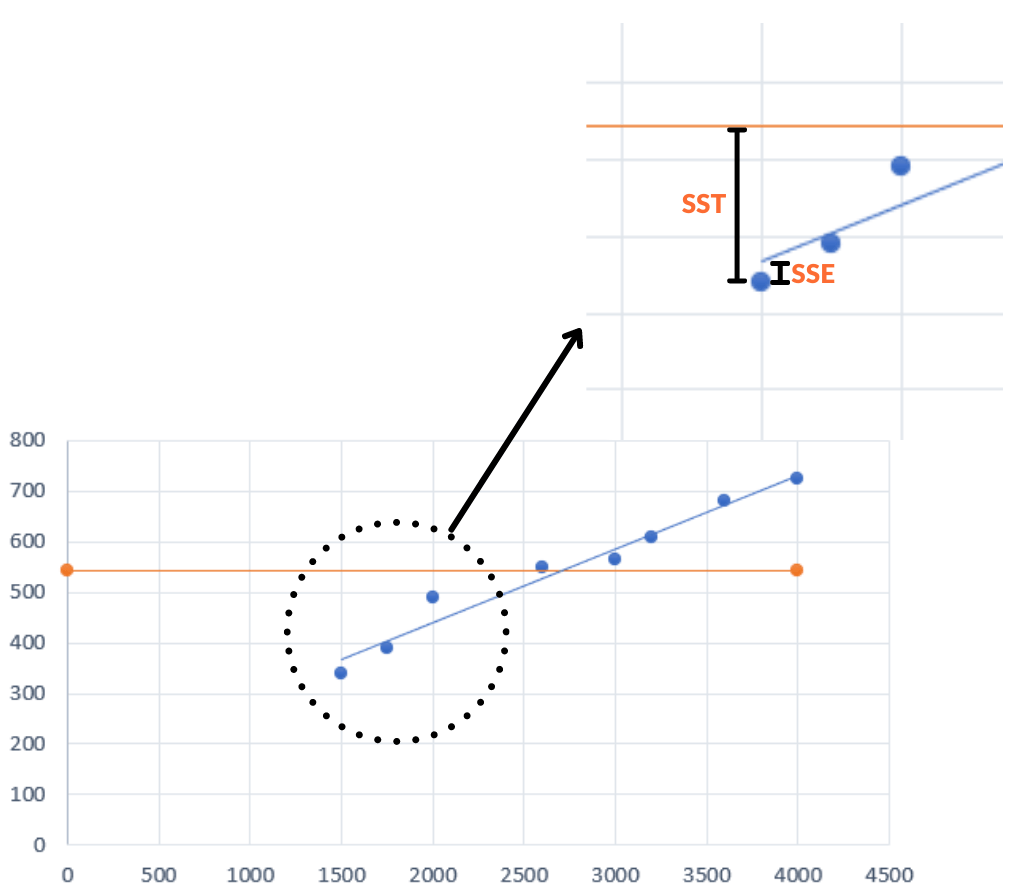Interpretation: In the above graph, observe how the distance from the line Ymean to the observed data point can be divided into exactly two distances. One is the SSE we discussed in the previous section and the second is the SSR.

Sum of Squared Residuals(SSR) is a statistical way to study the amount of variance in a regression model. It is the sum of squared values of residuals. Graphical interpretation will be –

SSR = SST – SSE

Here we have calculated SSE and now let’s calculate the Total Sum of Squared (SST). Consider the following diagram –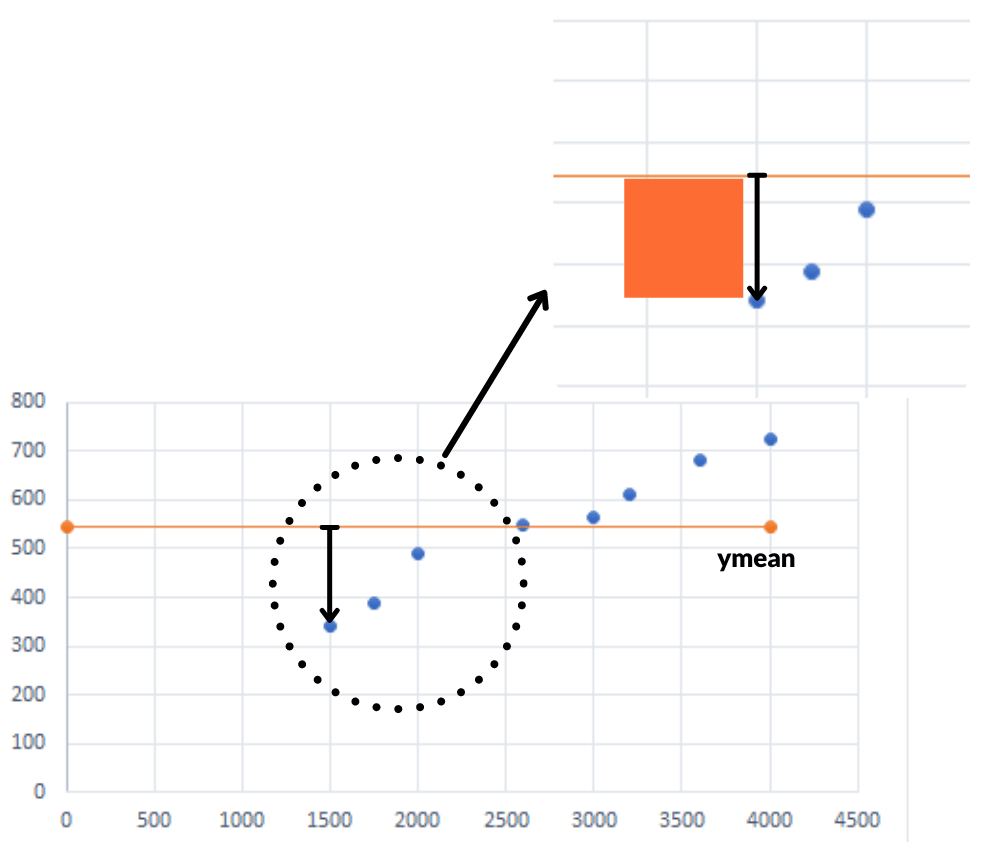Note: In this case, we considered only the dependent variable which is the price of the house. We figured out its mean and marked a horizontal line at $543.75. Squaring the distance between the observed data point to mainline and then adding them. Area (square feet) Price (thousand$)
Price difference wrt ymean
Squared
1500
340
-203.75
41514.0625
1750
390
-153.75
23639.0625
2600
550
6.25
39.0625
3000
565
21.25
451.5625
3200
610
66.25
4389.0625
3600
680
-136.25
18564.0625
4000
725
181.25
32851.5625
2000
490
-53.75
2889.0625

In the last column, on adding the values we get the Total Sum of Squares (SST).

Total Sum of Squares = SST =$\displaystyle\sum_{i=1}^n(Observed(dependent) -ymean)^2$ = 124337.5

Recall the relation between SSR, SSE, and SST.

SSR = SST – SSE = 124337.5 – 4464.5257 = 119872.9743

Thus, we can say that “Residual is the estimation of error”.

Coefficient of Determination: Now that we have values of SSR, SSE, and SST. Let’s go ahead and find the coefficient of determination represented as$R^2$. It is a statistical technique to measure the goodness of fit.

R-squared is generally interpreted as the percentage. If SSR is large, more SST is used and thus, SSE is smaller relative to the total. This ratio acts as a percentage.

Mathematically, it’s the sum of squares regression divided by the Total Sum of Squares.$R^2 = \frac{Sum of Squared Regression}{Total Sum of Squares} = \frac{SSR}{SST}$

Note-1: The value is always between 0(0%) and 1(100%).

Note-2: Larger values of$R^2$ suggest that our linear model is a good fit for the data we provided.

In our case,$R^2 = \frac{119872.9743}{124337.5} = 0.9641$ or 96.41%

Conclusion: We can conclude that 96.41% of the total sum of squares can be explained by our regression equation to predict house prices. Thus the error percentage is less than 4% which implies a GOOD FIT for our model.

While coding in S2, we include the following line to print the coefficient of determination.

				
println("R2: ${olsResiduals.R2()}",)   Mean Square Error: Represented as$s^2$ and tells us about how spread out the data points are from the regression line.$s^2$ is the estimate of sigma squared that is variance. So, MSE is SSE divided by its degrees of freedom which in our case is 2 because we are estimating slope and intercept. Note-1: In simple linear regression, the degree of freedom is always 2. Note-2: MSE is not simply the average of residuals.$s^2 = \frac{SSE}{n-2} = \frac{4464.5257}{6} = 744.08$ Standard Error: It is the standard deviation of the overall error. Represented as$s$. It is termed as the average distance an observation/data point falls from the regression line in units of the dependent variable.$s = \sqrt{MSE} = \sqrt{744.08} = 27.278$$s$ is a measure of how well the regression model makes prediction. When coding in S2, the following lines are typed printing –   println("standard error:${olsResiduals.stderr()}, f: ${olsResiduals.Fstat()}",) //standard error   Testing Data Implementation Consider the same question we coded previously in the “Building Regression Model” section. Here, we will predict values and find errors associated with the data. Consider the vector for testing as:$Y = \begin{bmatrix}1.2\\2.4\\4.1\\5.3\\9.9\end{bmatrix}$.   %use s2 val Y: Vector = DenseVector(arrayOf(1.0, 2.0, 4.0, 5.0, 10.0)) val X: Matrix = DenseMatrix( arrayOf( doubleArrayOf(25.0), doubleArrayOf(35.0), doubleArrayOf(60.0), doubleArrayOf(75.0), doubleArrayOf(200.0) ) ) val intercept = true val problem1 = LMProblem(Y, X, intercept) printOLSResults(problem1) // Testing data vector val W: Vector = DenseVector(arrayOf(1.2, 2.4, 4.1, 5.3, 9.9)) val problem2 = LMProblem(Y, X, intercept, W) printOLSResults(problem2) fun printOLSResults(problem: LMProblem?) { val ols = OLSRegression(problem) val olsResiduals: OLSResiduals = ols.residuals() println("beta hat:${ols.beta().betaHat()},\nstderr: ${ols.beta().stderr()}, \nresiduals:${olsResiduals.residuals()}")
println("R2: ${olsResiduals.R2()}, standard error:${olsResiduals.stderr()}, f: ${olsResiduals.Fstat()}",) println("fitted values:${olsResiduals.fitted()}")
println("sum of squared residuals: ${olsResiduals.RSS()}") println("total sum of squares:${olsResiduals.TSS()}")
println()
}




Output:

beta hat: [0.048918, 0.535481] ,
stderr: [0.005264, 0.532022] ,
residuals: [-0.758430, -0.247609, 0.529441, 0.795672, -0.319074]
R2: 0.9664281242711773, standard error: 0.7420099473407974, f: 86.36051188299813
fitted values: [1.758430, 2.247609, 3.470559, 4.204328, 10.319074]
sum of squared residuals: 1.6517362858580784
total sum of squares: 49.2

beta hat: [0.045201, 1.055123] ,
stderr: [0.003621, 0.504376] ,
residuals: [-1.185147, -0.637157, 0.232818, 0.554804, -0.095319]
R2: 0.9811093504747105, standard error: 1.23873309823374, f: 155.80872682454918
fitted values: [2.185147, 2.637157, 3.767182, 4.445196, 10.095319]
sum of squared residuals: 4.60337906597928
total sum of squares: 243.68558951965065

Outliers

We have now covered the most important part of regression. In this section, we will understand the concept of outliers.

Sometimes when you make a scatter plot, some data points or point just don’t look right. Consider the following scatter plot for example.Here, you will notice that in the above case the orange point looks way out of place. So, for one of the variables considered, a value can appear out of the norm. A point having such large residual values even though it is in the range of one variable is termed as an outlier.

Note: Outlier affects the slope of the regression line as it falls outside the general pattern of data.

We now conclude the topic, “Simple Linear Regression”.

Thank you for spending time on this course! Hope you learned a lot.